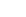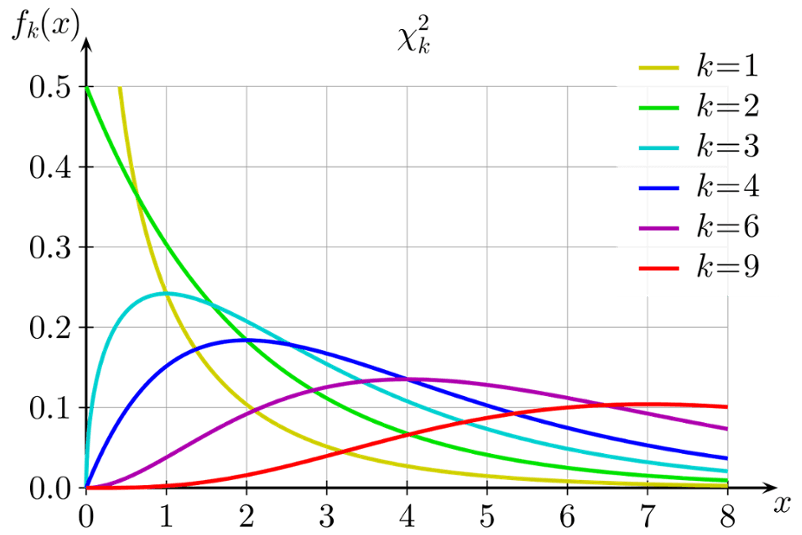Welcome to Innominds Blog
Enjoy our insights and engage with us!

Many a times, data scientists and analysts input data and then train a model like logistic regression for classification. Most of the practitioners do not seem to spend enough time on this part of the output and instead focus only on the top level diagnostics consisting of coefficient summary, RMSE or classification matrix and may be the overall measure such as an R2 in the case of a linear regression or a C-Stat or an area under curve (AUC) for logistic regression. While not denying the value of these top level diagnostics, it is also important to check for model fit than just the estimation or classification error. This is when the deviance based diagnostics come handy.Source: By Geek3 - Own work, CC BY 3.0, https://commons.wikimedia.org/w/index.php?curid=9884213

Considering the quartile distribution of the deviance residuals from the above tells us how the deviance residuals vary with each quartile and how they can be used to test the independence and normality (a critical assumption for linear regression) of the error terms whose distribution is unknown and can only be seen or approximated empirically by residuals. In this case, the quartile distribution of the deviance residuals suggests that there is a basis to reject the independence of the residuals and also a residual plot gives more information upon how the residuals are distributed.The Null deviance indicates whether the model with just the intercept explains better than the saturated model and the Residual deviance indicates whether the model with intercept and parameters explains better than the saturated model. These deviances fit to a Chi2 distribution and a check for the probability for 458.52 for 394 degrees of freedom is 0.013651 and for 499.98 on 399 degrees of freedom is 0.000428.

We can now conclude that the Null deviance and Residual deviance in this case are for real (since the probability values are less than the significance threshold value of 0.01). This implies that the proposed model explains significantly better than the saturated model.

Hence, whenever these deviance measures are seen; it is advisable to make use of the deviances and test your model fit much before you step to the output and diagnostics. There are more goodness of fit measures for advanced diagnostics and more of that later.

Topics: Big Data & Analytics, Technology### Ravi Kumar Meduri

Executive Vice President - Digital and IP Solutions